Courses

# Test: Linear Inequalities (CBSE Level) - 1

## 25 Questions MCQ Test Mathematics (Maths) Class 11 | Test: Linear Inequalities (CBSE Level) - 1

Description
This mock test of Test: Linear Inequalities (CBSE Level) - 1 for JEE helps you for every JEE entrance exam. This contains 25 Multiple Choice Questions for JEE Test: Linear Inequalities (CBSE Level) - 1 (mcq) to study with solutions a complete question bank. The solved questions answers in this Test: Linear Inequalities (CBSE Level) - 1 quiz give you a good mix of easy questions and tough questions. JEE students definitely take this Test: Linear Inequalities (CBSE Level) - 1 exercise for a better result in the exam. You can find other Test: Linear Inequalities (CBSE Level) - 1 extra questions, long questions & short questions for JEE on EduRev as well by searching above.
QUESTION: 1

Solution:
QUESTION: 2

Solution:
QUESTION: 3

### Identify the solution set for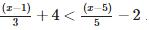Solution:
QUESTION: 4

What is the solution set for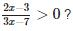Solution:
QUESTION: 5

Identify the solution set for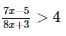Solution:

(7x-5)/(8x+3) > 4
(7x-5)/(8x+3) - 4 >0
7x - 5 - 4 ( 8x + 3 ) / 8x + 3 > 0
- 25 x - 17 / 8x + 3 > 0
Now furthermore solving for general range :
x ∈ ( -17/ 25, - 3/8)

QUESTION: 6

What is the solution set for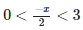Solution:
QUESTION: 7

Identify solution set for | 4 − x | + 1 < 3?

Solution:

|4 − x| + 1 < 3
⇒ 4 − x + 1 < 3
Add −4 and −1 on both sides, we get
4 − x + 1 − 4 − 1 < 3 − 4 − 1
⇒ − x < −2
Multiply both sides by −1, we get
x > 2
Also,|4−x| + 1 < 3
⇒ −(4−x) + 1 < 3
⇒ − 4 + x + 1 < 3
Add 4 and −1 on both sides, we get
− 4 + x + 1 + 4 − 1 < 3 + 4 − 1
⇒ x < 6
Thus, x ∈ (2,6).

QUESTION: 8

What is the solution set for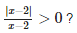Solution:

|x-2|/(x-2) > 0
=> x - 2 > 0
x > 2
x denotes (2,∞)

QUESTION: 9

Identify the solution set for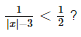Solution:

Explanation:- 1/|x| - 3 < ½

By cross multiply, we get

2 > |x| - 3

2+3 <|x|

5 < |x|

(5,∞)

QUESTION: 10

What is the solution set for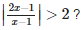Solution:

|(2x-1)/(x-1)| > 2
|x| > a
⇒ x > a
or x < -a
(2x-1)/(x-1) > 2 and (2x-1)/(x-1) < -2
(2x-2+1)/(x-1) > 2
⇒ (2(x-1) + 1)/(x-1) > 2
⇒ 2 + (1/(x-1)) > 2
1/(x-1) > 0
x-1 < 0
x < 1...........(1)
Now taking, (2x-1)/(x-1) < -2
2 + (1/(x-1) < -2
= 1/(x-1) < -4
x-1 > -1/4
x > -1/4 + 1
x > 3/4.......(2)
From (1) and (2)
x implies (3/4, 1)∪ (⁡1,∞)

QUESTION: 11

In the first four papers each of 100 marks, Rishi got 95, 72, 73, 83 marks. If he wants an average of greater than or equal to 75 marks and less than 80 marks, find the range of marks he should score in the fifth paper .

Solution:

Let marks in 5th paper be x
Average = (95 + 72 + 73 + 53 + x)/5
= (323 + x)/5
(75 ≤ 323 + x)/5 < 80
375 ≤ 323 + x < 400
52 ≤ x < 77
[52,77).

QUESTION: 12

A man wants to cut three lengths from a single piece of board of length 91cm. The second length is to be 3 cm longer than the shortest and third length is to be twice as long as the shortest. What are the possible lengths for the shortest board if the third piece is to be at least 5 cm longer than the second?

Solution:
QUESTION: 13

Find all pairs of consecutive odd natural numbers, both of which are larger than 10, such that their sum is less than 40.

Solution:
QUESTION: 14

The marks scored by Rohit in two tests were 65 and 70. Find the minimum marks he should score in the third test to have an average of atleast 65 marks.

Solution:
QUESTION: 15

A solution is to be kept between 30C and 35C What is the range of temperature in degree Fahrenheit ?

Solution:
QUESTION: 16

The longest side of a triangle is three times the shortest side and the third side is 2cm shorter than the longest side if the perimeter of the triangles at least 61cm, find the minimum length of the shortest side.

Solution:
QUESTION: 17

Which of the following is correct ?

Solution:
QUESTION: 18

Solve the inequality 3 − 2x ≤ 9

Solution:

3 − 2x ≤ 9
-2x ≤ 6
-x ≤ 3
-3 ≤ x

QUESTION: 19

Given that x is an integer, find the values of x which satisfy both 2x + 3 > 7 and x + 4 < 10

Solution:
QUESTION: 20

The solution of 4x-2 > 6 is

Solution:
QUESTION: 21

x = 4 , 5 and 6 are the solutions for :

Solution:
QUESTION: 22

What are the integer values of x which satisfy the inequalities x > − 2 and x ≤ 2 ?

Solution:
QUESTION: 23

Given that x is an integer, find the values of x which satisfy the simultaneous linear inequalities 2 + x < 6 and 2 −3x < − 1.

Solution:
QUESTION: 24

Solve : 30x < 200, when x is a natural number :

Solution:
QUESTION: 25

The solution set for : 3x − 7 > x + 3.

Solution: